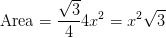# SSAT Upper Level Math : How to find the area of an equilateral triangle

## Example Questions

### Example Question #9 : Equilateral Triangles

An equilateral triangle is circumscribed about a circle of radius 16. Give the area of the triangle.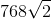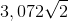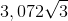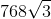The correct answer is not among the other choices.Explanation:

The circle and triangle referenced are below, along with a radius to one side and a segment to one vertex: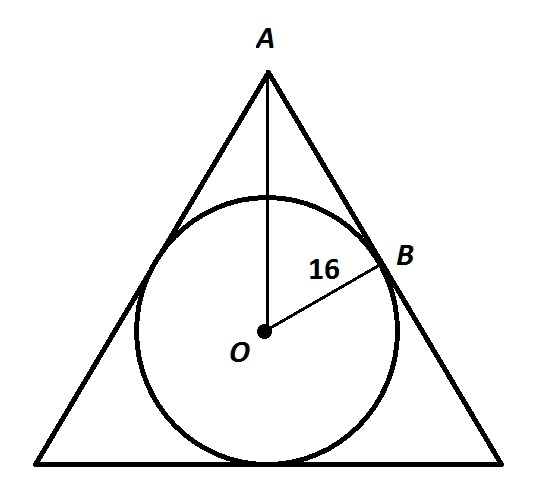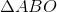is a 30-60-90 triangle, so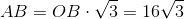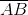is one-half of a side of the triangle, so the sidelength is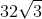. The area of the triangle is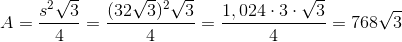### Example Question #10 : Equilateral Triangles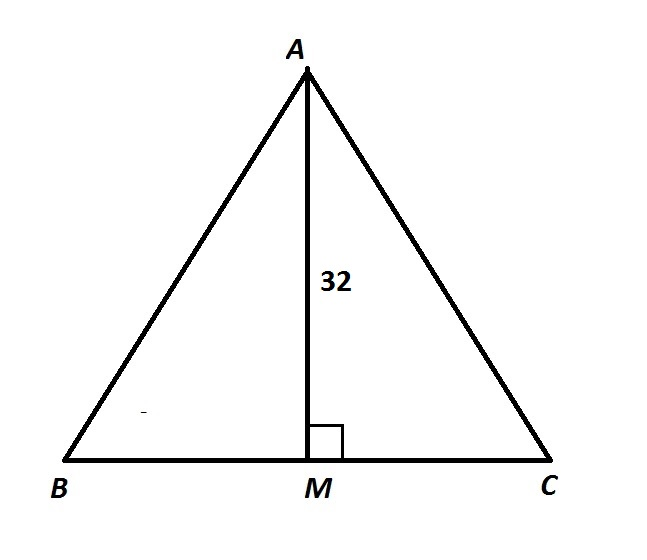In the above diagram,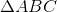is equilateral. Give its area.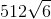The correct answer is not among the other responses.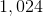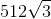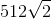The correct answer is not among the other responses.

Explanation:

The interior angles of an equilateral triangle all measure 60 degrees, so, by the 30-60-90 Theorem,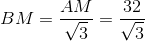Also,is the midpoint of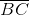, so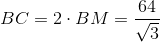; this is the base.

The area of this triangle is half the product of the base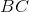and the height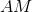: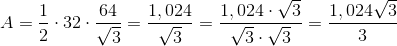This answer is not among the given choices.

### Example Question #1 : How To Find The Area Of An Equilateral Triangle

The perimeter of an equilateral triangle is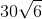. Give its area.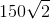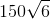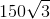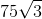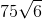Explanation:

An equilateral triangle with perimeterhas three congruent sides of length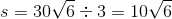The area of this triangle is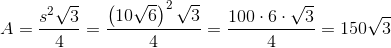### Example Question #2 : How To Find The Area Of An Equilateral Triangle

Hexagon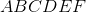is regular and has perimeter 72.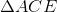is constructed. What is its area?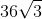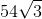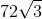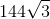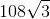Explanation:

Since the perimeter of the (six-congruent-sided) regular hexagon is 72, each side has length one sixth this, or 12.

The figure described is given below, with a perpendicular segment drawn fromto side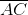: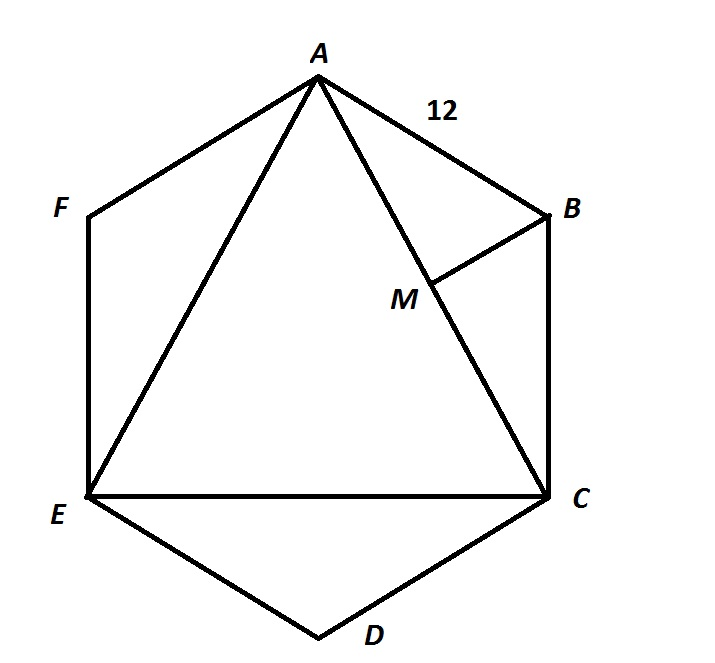Each angle of a regular hexagon measures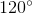. Therefore,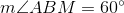, and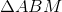is a 30-60-90 triangle.

By the 30-60-90 Theorem,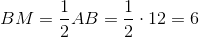and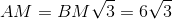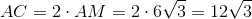.is equilateral, and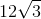is its sidelength, making its area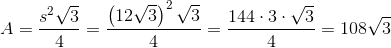### Example Question #3 : How To Find The Area Of An Equilateral Triangle

The perimeter of an equilateral triangle is. Give its area.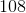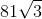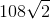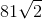Explanation:

An equilateral triangle with perimeter 54 has three congruent sides of length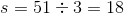The area of this triangle is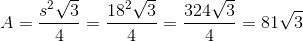### Example Question #4 : How To Find The Area Of An Equilateral Triangle

An equilateral triangle is inscribed inside a circle of radius. Give the area of the triangle.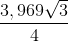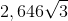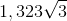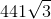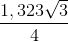Explanation:

The trick is to know that the circumscribed circle, or the circumcircle, has as its center the intersection of the three altitudes of the triangle, and that this center, or circumcenter, divides each altitude into two segments, one twice the length of the other - the longer one being a radius. Because of this, we can construct the following: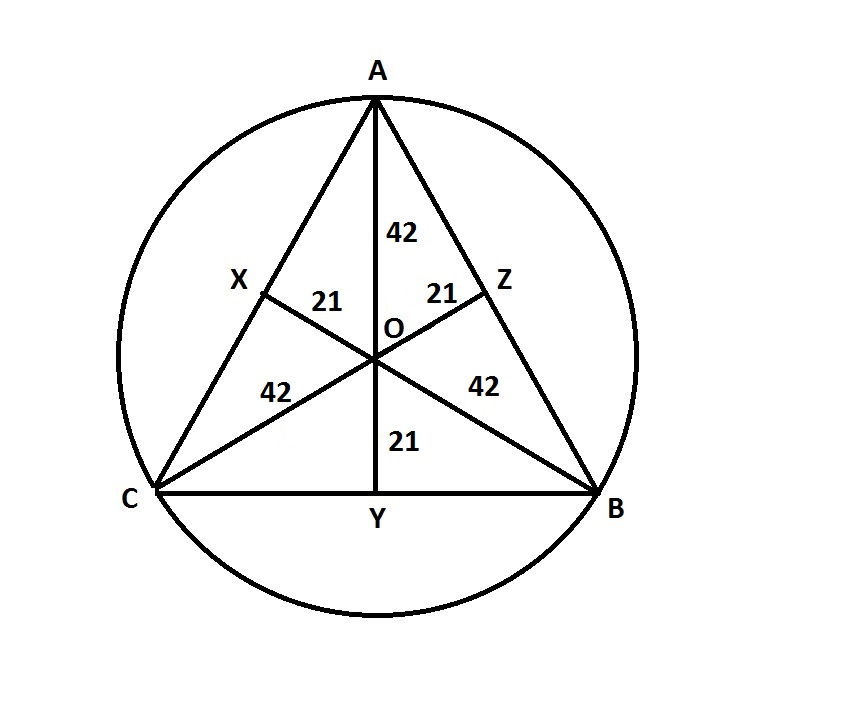Each of the six smaller triangles is a 30-60-90 triangle, and all six are congruent.

We will find the area of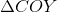, and multiply it by 6.

By the 30-60-90 Theorem,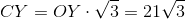, so the area ofis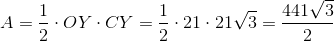.

Six times this -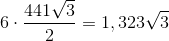- is the area of.

### Example Question #5 : How To Find The Area Of An Equilateral Triangle

An equilateral triangle has side lengths of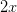. What is the area of this triangle?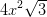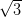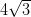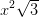Explanation:

The area of an equilateral triangle can be found using this formula: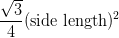Using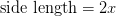, we can find the area of the equilateral triangle.